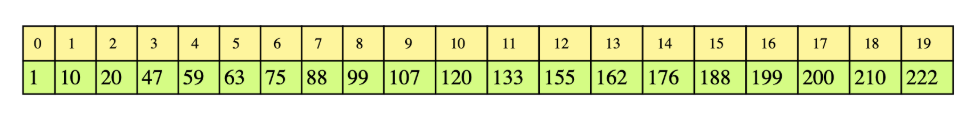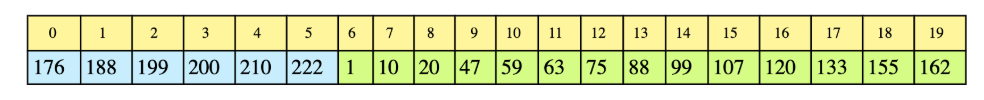# Search Rotated Array

## Problem Statement

Search for a given number in a sorted array, with unique elements, that has been rotated by some arbitrary number. Return -1 if the number does not exist.

Assume that the array does not contain duplicates

Below is an original array before rotationAfter performing rotation on this array 6 times it changes to:The task is to find a given number in this array.

## Hint

• Linear search is not an acceptable solution
• Try to solve the problem using binary search

## Try it yourself

int binary_search_rotated(vector<int>& arr, int key) {  // TODO: Write - Your - Code  return -1;}

## Solution

int binary_search(vector<int>& arr, int start, int end, int key) {  // assuming all the keys are unique.    if (start > end) {    return -1;  }  int mid = start + (end - start) / 2;  if (arr[mid] == key) {    return mid;  }  if (arr[start] <= arr[mid] && key <= arr[mid] && key >= arr[start]) {    return binary_search(arr, start, mid-1, key);  }  else if (arr[mid] <= arr[end] && key >= arr[mid] && key <= arr[end]) {    return binary_search(arr, mid+1, end, key);  }  else if (arr[end] <= arr[mid]) {    return binary_search(arr, mid+1, end, key);  }  else if (arr[start] >= arr[mid]) {    return binary_search(arr, start, mid-1, key);  }  return -1;}int binary_search_rotated(vector<int>& arr, int key) {  return binary_search(arr, 0, arr.size()-1, key);}int main(int argc, char* argv[]) {    vector<int> v1 = {6, 7, 1, 2, 3, 4, 5};      cout<<"Key(3) found at: "<<binary_search_rotated(v1, 3)<<endl;    cout<<"Key(6) found at: "<<binary_search_rotated(v1, 6)<<endl;        vector<int> v2 = {4, 5, 6, 1, 2, 3};        cout<<"Key(3) found at: "<<binary_search_rotated(v2, 3)<<endl;    cout<<"Key(6) found at: "<<binary_search_rotated(v2, 6)<<endl;    }

## Solution Explanation

### Runtime complexity

The runtime complexity of this solution is logarithmic, O(log n).

### Memory complexity

The memory complexity of this solution is logarithmic, O(log n).

### Solution Breakdown

The solution is essentially a binary search but with some modifications. If we look at the array in the example closely, we notice that at least one half of the array is always sorted. We can use this property to our advantage. If the number ‘n’ lies within the sorted half of the array, then our problem is a basic binary search. Otherwise, discard the sorted half and keep examining the unsorted half. Since we are partitioning the array in half at each step, this gives us O(log n) runtime complexity.

Practice problems like this and many more by checking out our Grokking the Coding Interview: Patterns for Coding Questions course!

Learn in-demand tech skills in half the time